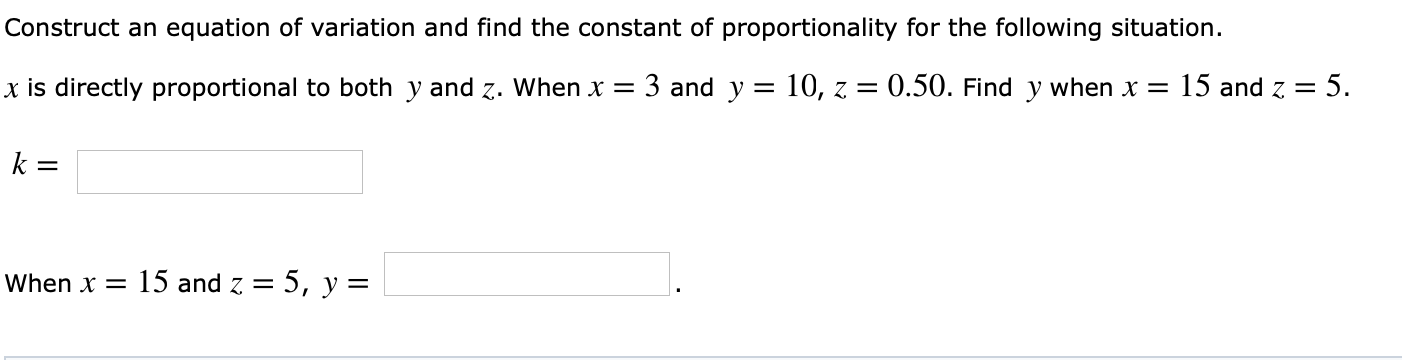# Construct an equation of variation and find the constant of proportionality for the following situation. x is directly proportional to both y and z. Whenx 3 and y -10, z0.50. Find y when x 15 and z 5 When 15 and z-5, y-

Questionhelp_outlineImage TranscriptioncloseConstruct an equation of variation and find the constant of proportionality for the following situation. x is directly proportional to both y and z. Whenx 3 and y -10, z0.50. Find y when x 15 and z 5 When 15 and z-5, y- fullscreen

### Want to see this answer and more?

Experts are waiting 24/7 to provide step-by-step solutions in as fast as 30 minutes!*